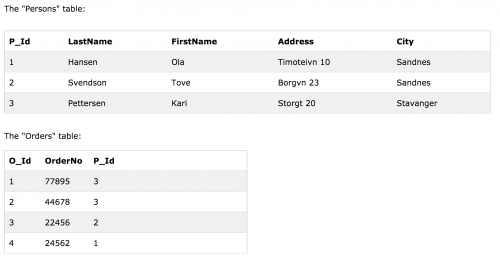# Zend PHP 7 Certification – Databases – SQL

This post covers the SQL section of the Databases chapter when studying for the Zend PHP 7 Certification.

SQL is a standard language used for accessing and manipulating databases. Within a database there consists one of more tables.

A primary key is a field in a table which uniquely identifies each row/record in a database table. Primary keys must contain unique values. A primary key column cannot have NULL values.

Most tables should have a primary key, and each table can have only ONE primary key. The primary key is usually the ‘ID’ column of database tables, such as `person_id` for a `Person` table, `order_id` for an `Order` table and so on.

A foreign KEY in one table points to a PRIMARY KEY in another table and therefore enables relational databases. As common practice is not to have all data in one table, but multiple tables, foreign keys are used to establish relationships between tables. Examples of this could be a `persons` table, and an `orders` table where the relationship is establishing which order associates to which person.The FOREIGN KEY constraint is used to prevent actions that would destroy links between tables.

So to create a table within MySQL, the following syntax can be used.

```CREATE TABLE tbl (
id INT NOT NULL PRIMARY KEY,
field1 VARCHAR(100),
field2 VARCHAR(100) NOT NULL
)
```

Note that `NULL` is not the same as `0` or `false`. It represents ‘no value’ or ‘missing value’.

To read the data from the database table, we can write a `SELECT` statement.

`SELECT field1, field2 FROM tbl WHERE field2 = 'somevalue'`

To insert data, use an `INSERT` statement.

```INSERT INTO tbl (field1, field2) VALUES (99, 'somevalue')

Likewise to update data, use an <code>UPDATE</code> statement.

UPDATE tbl SET field1 = 'somevalue', field2 = 'test123' WHERE id = 1```

And to delete data, use `DELETE`.

`DELETE FROM tbl WHERE id = 3`

Note that you can also use truncate and drop statements to empty and delete tables respectively.

```TRUNCATE TABLE tbl
DROP TABLE tbl
DROP DATABASE database1
```

To sort (order) data, we can write the following.

`SELECT * FROM tbl ORDER BY field1 DESC`

Sometimes, rather than retrieving individual records, you want to know something about a group of records. The `GROUP BY` clause is used here.

`SELECT * FROM tbl GROUP BY field1`

SQL aggregate functions return a single value, calculated from values in a column. Useful aggregate functions include the following.

• `AVG()`
• `COUNT()`
• `DISTINCT COUNT()`
• `MIN()`
• `MAX()`
• `SUM()`